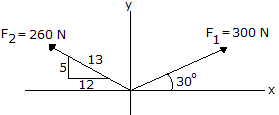# Engineering Mechanics - Force Vectors - Discussion

### Discussion :: Force Vectors - General Questions (Q.No.16)

16.Determine the magnitude and direction of the resultant force.

 [A]. R = 251 N,= 85.5° CCW [B]. R = 251 N,= 94.5° CCW [C]. R = 421 N,= 67.7° CCW [D]. R = 421 N,= 112.3° CCW

Explanation:

No answer description available for this question.

 Monica Ann said: (Jun 18, 2011) First, solve for the angle of F2. Let's have the angle as angle α (alpha). That could be solved by using the sine law. 13/sin90= 5/sinα Equating this gives us, α=22.61986495 degrees Now, we can solve for F1x, F1y, F2x, F2y, Rx, Ry, R (resultant) and θ. F1x= 300cos30 F1x= 259.8076211 N to the right F1y= 300sin30 F1y= 150 N, up F2x= -260cos22.61986495 F2x= 240 N to the left F2y= 260sin22,61986495 F2y= 100 N, up Rx= F1x+F2x = [259.8076211 + (-240)]N *Note that we have -F2x since the force is to the left. Rx= 19.80762114 N Ry= F1y + F2y = [150 + 100]N Ry= 250 N R=*squareroot of [Rx*squared + Ry*squared] =*squareroot of [(19.80...)*squared + (250)*squared] =*squareroot of (392.3418552 + 62500) =*squareroot of (62892.34186) R=250.7834561 N, which could be rounded to 251 N. θ= arctan (Ry/Rx) = arctan (250/19.80...) = arctan (12.62140457) θ= 85.46989102 degrees, which could be rounded to 85.5 degrees. Answer: 251 N, θ=85.5 degrees.

 Khuliso said: (Sep 12, 2011) The calculation are good, but is the any way we can do this?

 Rye said: (Jan 10, 2013) Solving for the component of x We have, fx = [300cos30]+[-260x12/13] fx = 20 N Solving for fy: fy=[300sin30]+[260x5/13] fy=250 N Therefore R=20squared+250square then taking its squareroot R=251 N. Solving for angle: Angle =arctan fy/fx Therefore: Angle=arctan250/20. Angle=85.5.

 Yoseph said: (Dec 27, 2013) Clear but if it will be in parallelogram.